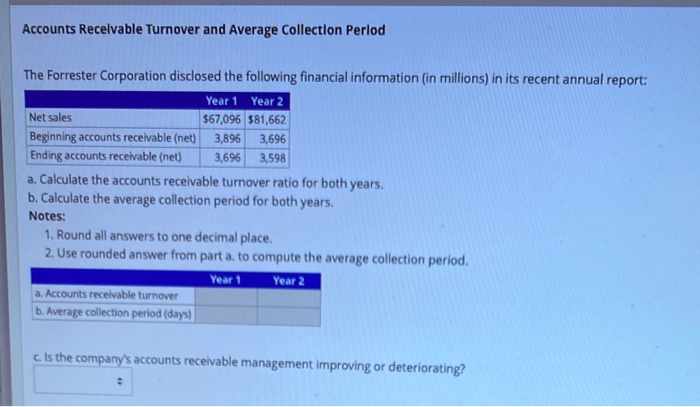# Question Accounts Receivable Turnover and Average Collection Period The Forrester Corporation disclosed the following financial information (in millions) in its recent annual report: Year 1 Year 2 Net sales $67,096$81,662 Beginning accounts receivable (net) 3,896 3,696 Ending accounts receivable (net) 3,696 3,598 a. Calculate the accounts receivable turnover ratio for both years. b. Calculate the average collection period for both years. Notes: 1. Round all answers to one decimal place. 2. Use rounded answer from part a. to compute the average collection period. Year 1 Year 2 a. Accounts receivable turnover b. Average collection period (days) c. Is the company's accounts receivable management improving or deteriorating?Transcribed Image Text: Accounts Receivable Turnover and Average Collection Period The Forrester Corporation disclosed the following financial information (in millions) in its recent annual report: Year 1 Year 2 Net sales $67,096$81,662 Beginning accounts receivable (net) 3,896 3,696 Ending accounts receivable (net) 3,696 3,598 a. Calculate the accounts receivable turnover ratio for both years. b. Calculate the average collection period for both years. Notes: 1. Round all answers to one decimal place. 2. Use rounded answer from part a. to compute the average collection period. Year 1 Year 2 a. Accounts receivable turnover b. Average collection period (days) c. Is the company's accounts receivable management improving or deteriorating?
Transcribed Image Text: Accounts Receivable Turnover and Average Collection Period The Forrester Corporation disclosed the following financial information (in millions) in its recent annual report: Year 1 Year 2 Net sales $67,096$81,662 Beginning accounts receivable (net) 3,896 3,696 Ending accounts receivable (net) 3,696 3,598 a. Calculate the accounts receivable turnover ratio for both years. b. Calculate the average collection period for both years. Notes: 1. Round all answers to one decimal place. 2. Use rounded answer from part a. to compute the average collection period. Year 1 Year 2 a. Accounts receivable turnover b. Average collection period (days) c. Is the company's accounts receivable management improving or deteriorating?Year 1:-{:[" Average Accounts Receivable=(Beginning Accounts Receivable+Ending Accounts Receivables)/2 "],[=($3","896+$3","696)//2=$7","592//2],[=$3","796]:}Accounts Receivables Turnover Ratio=Net Sales/Average Accounts Receivables=$67,096//$3,796=17.7" Times. "Average Collection Period=365 Days/Accounts Receivable Turnover Ratio=365//17.7=20.62" days "Year 2:-Average Accounts Receivable=(Beginning Accounts Receivable+Ending Accounts Receivables)/2{:[=($3","696+$3","59 ... See the full answer